Filters
Latest Questions
CBSE
Mathematics
Similarity of triangles
$\vartriangle ABC$ is similar to $\vartriangle PQR$. $AB$ corresponds to $PQ$ and $BC$ corresponds to $QR$. If $AB = 9$, $BC = 12$, $CA = 6$ and $PQ = 3$, what are the lengths of $QR$ and $RP$?
CBSE
Mathematics
Similarity of triangles
A vertical stick $20{\text{ m}}$ long casts a shadow $10{\text{ m}}$ long on the ground, at the same time a tower casts a shadow $50{\text{ m}}$ long on the ground, the height of the tower is?
A) $100{\text{ m}}$
B) $120{\text{ m}}$
C) $25{\text{ m}}$
D) $200{\text{ m}}$
CBSE
Mathematics
Similarity of triangles
Given, $D$ is the midpoint of side $BC$ of a $\vartriangle ABC$ . $AD$ is bisected at the point $E$ and $BE$ produces cuts $AC$ at the point $X$ . Prove that $BE:EX = 3:1$ .
CBSE
Mathematics
Similarity of triangles
In the given figure,find the value of $x$ in terms of $a$,$b$ and $c$.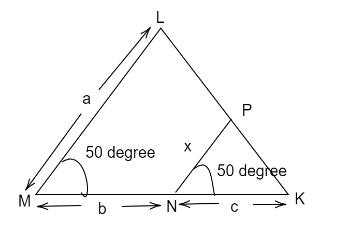CBSE
Mathematics
Similarity of triangles
In a $\vartriangle PQR$ , $P{R^2} - P{Q^2} = Q{R^2}$ and $M$ is a point on side $PR$ such that $QM \bot PR$ , prove that $Q{M^2} = PM \times MR$ .

CBSE
Mathematics
Similarity of triangles
In given figure , if DE|| BC, then the value of x is equal to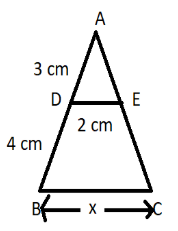(A). 3 cm
(B). 4 cm
(C). 7 cm
(D). 4.7 cm

CBSE
Mathematics
Similarity of triangles
If two triangles are similar, then the ratio of the area of both triangles is proportional to the square of the ratio of their corresponding sides.

CBSE
Mathematics
Similarity of triangles
Given $area\left( \Delta ABC \right)=360c{{m}^{2}},area\left( BPQC \right)=110c{{m}^{2}}$, $PQ||BC;AP=10cm$. Find $\dfrac{AQ}{QC}$.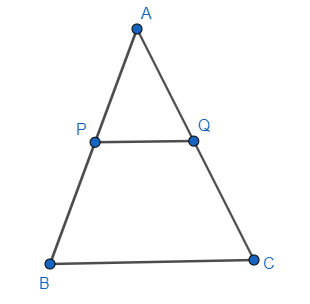CBSE
Mathematics
Similarity of triangles
If two triangles $\Delta DEF\sim \Delta PQR$. If 2DE = 3PQ, QR = 8 and DF = 6 then find EF and PR?
CBSE
Mathematics
Similarity of triangles
If similar triangles, $\Delta ABC\sim \Delta DEF$ in which $AH$ and $DY$ are the bisectors of $\angle A$ and $\angle D$ respectively. If $AH=6.5\,\text{ cm}$ and $DY=5.2\text{ cm}$ , find the ratio of the area of $\Delta ABC$ and $\Delta DEF$ .
CBSE
Mathematics
Similarity of triangles
In the figure $\angle M=\angle N={{46}^{\circ }}$, express $x$ in terms of a, b and c where lengths of LM, MN and NK are a, b, c respectively. $PN=x$.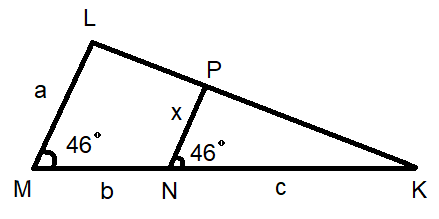CBSE
Mathematics
Similarity of triangles
In quadrilateral $ACBD$,
$AC = AD$ and $AB$bisects $\left| \!{\underline {\, A \,}} \right.$. Show that $\Delta \;ABC \cong \Delta \;ABD$. What can you say about $BC$and $BD$ $?$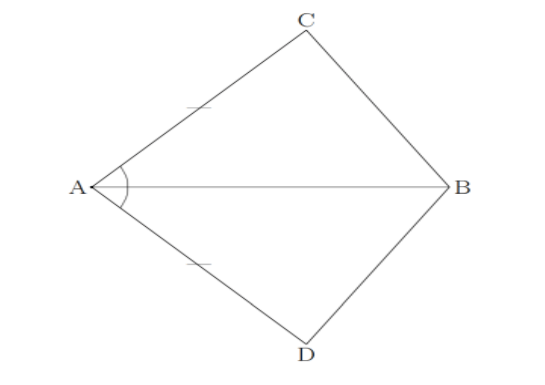Prev
1
2
3
4
5